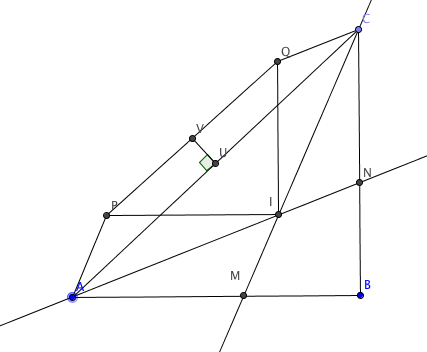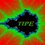# Right triangle and two parallelogramsThis is the first problem of #PeruMOTraining, you can see my first post here. I proposed this problem for the Peruvian Mathematical Olympiad, in 2011. Please post your solutions!

Problem Let $ABC$ be a right triangle, with $\angle ABC=90^\circ$. Let $CM$ and $AN$ be interior bisectors intersecting at $I$ ($M$ is on the segment $AB$ and $N$ is on the segment $BC$ ). Construct the paralellograms $AMIP$ and $CNIQ$. If $U$ and $V$ are the midpoints of segments $AC$ and $PQ$, respectively. Prove that $UV$ and $AC$ are perpendicular.Note by Jorge Tipe
6 years, 11 months ago

This discussion board is a place to discuss our Daily Challenges and the math and science related to those challenges. Explanations are more than just a solution — they should explain the steps and thinking strategies that you used to obtain the solution. Comments should further the discussion of math and science.

When posting on Brilliant:

• Use the emojis to react to an explanation, whether you're congratulating a job well done , or just really confused .
• Ask specific questions about the challenge or the steps in somebody's explanation. Well-posed questions can add a lot to the discussion, but posting "I don't understand!" doesn't help anyone.
• Try to contribute something new to the discussion, whether it is an extension, generalization or other idea related to the challenge.

MarkdownAppears as
*italics* or _italics_ italics
**bold** or __bold__ bold
- bulleted- list
• bulleted
• list
1. numbered2. list
1. numbered
2. list
Note: you must add a full line of space before and after lists for them to show up correctly
paragraph 1paragraph 2

paragraph 1

paragraph 2

[example link](https://brilliant.org)example link
> This is a quote
This is a quote
    # I indented these lines
# 4 spaces, and now they show
# up as a code block.

print "hello world"
# I indented these lines
# 4 spaces, and now they show
# up as a code block.

print "hello world"
MathAppears as
Remember to wrap math in $$ ... $$ or $ ... $ to ensure proper formatting.
2 \times 3 $2 \times 3$
2^{34} $2^{34}$
a_{i-1} $a_{i-1}$
\frac{2}{3} $\frac{2}{3}$
\sqrt{2} $\sqrt{2}$
\sum_{i=1}^3 $\sum_{i=1}^3$
\sin \theta $\sin \theta$
\boxed{123} $\boxed{123}$

Sort by:

Let's assume AB=a, BC=b and CA=c. X is the point so that VX is parallel to AB and UX is parallel to BC. So VXU is a right triangle at X. Let r be the inradius of ABC, so r = (a+b-c)/2. Let's calculate VX and UX.

VX = (1/2)IP + r - (1/2)AB = (1/2) (AM + 2r - a) = (1/2) (AM + (b-c))

By the angle bisector theorem AM/MB = c/b, so AM/a=c/(b+c), or AM = ac/(b+c) . Hence

(b+c)(AM + (b-c)) = ac + (b^2-c^2) = ac - a^2 = a(c-a) = a(c-a)(c+a)/(a+c) = ab^2/(a+c)

So 2(a+c)(b+c)VX = ab^2 Similarly 2(a+c)(b+c)UX = ba^2

So VX/UX= b/a = CB/AB. Hence VXU is similar to CBA. This should be enough to conclude UV is perpendicular to AC.

- 6 years, 11 months ago

Please, can you explain why $VX= (1/2)IP + r - (1/2)AB$ ?

- 6 years, 11 months ago

The (distance from V to BC) is the (distance from V to IQ) + the (distance from I to BC)

The (distance from V to IQ) = (1/2)IP

The (distance from I to BC) = r

The (distance from U to BC) = (1/2)AB

VX = (distance from V to BC) - (distance from U to BC)

- 6 years, 11 months ago

Wow I'm so happy that the new features have given us not only geometry problems, but also more discussions.

This is a very nice problem here I will give a synthetic solution:

Let's take advantage of all these midpoints. We construct a point on ray$AV$ such that $V$ is the midpoint of $AK$.Now we have $VU\parallel CK$, which means we just have to prove $CK\perp AC$. Moreover, we get parallelogram $APKQ$ so $QK=MI, IN=QC, \angle KQC=\angle CIN=45$. Now since $\angle QCA=\angle CAN=\angle NAB$, therefore $CK\perp AC$ is equivalent to proving $\angle KCQ=90-\angle QCA=90-\angle NAB=\angle ANB$. Since $\angle KQC=45=\angle NBI$, thus we just have to prove $\triangle KQC\sim \triangle IBN$ or simply $\frac {BI}{BN}=\frac {QK}{QC}=\frac {MI}{IN}$. This is indeed true from $\triangle MIB\sim \triangle INB$ and here's its proof: since $\angle AIM=\angle ABI=45$, therefore $\angle NIB=\angle IAM+\angle IBA=\angle IAM+\angle AIM=\angle IMB$. It's already known that $\angle IBM=\angle IBN$, so that gives us the similarity. $\Box$

I haven't been keeping up with all these posts lately, wish I had found this sooner. :)

- 6 years, 11 months ago

Nice solution Xuming! You find another property of a right triangle: triangles $MBI$ and $IBN$ are similar!

- 6 years, 11 months ago

I actually have a few more. I found them while trying different constructions of points and lines.

- 6 years, 11 months ago

The following is a nice property about a right triangle.

[Keeping the same notation of our problem]

Property
The projections of the segments $MI$ and $IN$ on the line $AC$ have the same length.

Proof Let $E$ and $F$ in $AC$ such that $ME$ and $NF$ are perpendicular to $AC$. Denoting $d(X,YZ)$ the distance from point $X$ to line $YZ$, we have $d(I, ME)=d(I,MB)=r=d(I, NB)=d(I,NF)$ . Since $d(I,ME)$ is the length of the projection of $MI$ on the line $AC$ and $d(I,NF)$ is the length of the projection of $NI$ on the line (AC), we are done!

Could you use this property to solve our problem?

- 6 years, 11 months ago

OK, so if X, Y are on AC such that both PX and QY are perpendicular to AC, what you proved above implies that AX=CY as both are projections of AP and CQ onto AC. Hence U is the midpoint of XY. Since V is the midpoint of PQ, VU is parallel to PX. Hence VU is perpendicular to AC.

- 6 years, 11 months ago

The lemma seems to be unnecessary. Let X and Y as above, then $\angle{PAX}=\angle{ACM} =\frac{C}{2}$. So $AX = AP\cos{\frac{C}{2}} = IM\cos{\frac{C}{2}} =r$. Similarly $CY=r$. The rest is the same as above.

- 6 years, 11 months ago

Basically the lemma states that $AX=CY$. My idea was to stand out a property concerning the points M, I, N without mention the other points.

- 6 years, 11 months ago

Yes, Thank you. A proof along that line was not possible for me without seeing your lemma.

- 6 years, 11 months ago

Are we allowed to use co-ordinate geometry? I thought of a solution using co-ordinate geometry but it is turning out to be a bit ugly.

- 6 years, 11 months ago

I believe so. All solutions are welcome.

- 6 years, 11 months ago

Since we have a right angle, a solution with co-ordinate geometry seems possible.

- 6 years, 11 months ago

Yes, I did with coordinates too, finding the coordinates of V is pretty much same as what I posted above. Then it's just a matter of verifying the slope of VU is the negative reciprocal of the slope of AC.

- 6 years, 11 months ago

Please post a solution that is understandable and graceful......... I'm still in Middle School

- 6 years, 11 months ago

AC is parallel PQ UV &AC are perpendicular

- 6 years, 11 months ago

In general, $AC$ and $PQ$ are not parallel.https://i.imgur.com/1RSGjPt.png

- 6 years, 11 months ago

Even AC and PQ are parallel can't conclude that VU and AC are perpendicular unless AP=CQ which is clearly not true.

- 6 years, 11 months ago

And AP=CQ implies that it's a 45-45-90 triangle... I'm posting this so we can have 3 people from San Diego replying to the same post. :) Such a rare scene.

- 6 years, 11 months ago

Best and shortest solution here I think.

- 6 years, 11 months ago